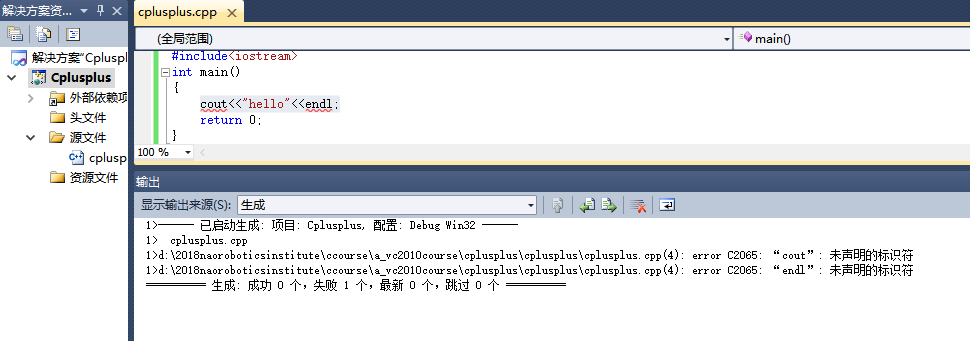# C++命名空间namspace解析——“cout”未声明的标识符，“cin”未声明的标识符

#include<iostream>
int main()
{

return 0;
}cout<<"hello"<<endl;


using namespace std;#include<iostream>
int main()
{
std::cout<<"hello"<<std::endl;
return 0;
}


#include<iostream>
using namespace std;
namespace A{
int x=1;
void f(){
cout<<"A"<<endl;
}
}
namespace B{
int x=2;
void f(){
cout<<"B"<<endl;
}
}
int main()
{
cout<<A::x<<endl;
B::f();
return 0;
}


1
B

namespace实例
#include <iostream>
#include <stdlib.h>
using namespace std;
namespace   sp            //填写命名空间的关键字
{
int x = 105;
}
int main()
{
// 使用bool类型定义isOdd，作为状态位
bool isOdd ;

if(sp::x % 2 == 0)
{
//改变状态位的值，使其为false
isOdd=false;
}
else
{
//改变状态位的值，使其为true
isOdd = true;
}
// 判断状态位的值
if(isOdd==true)
{
// 如果状态位的值为true，则打印变量x是奇数
cout<<"变量x是奇数"<<endl;
}
else
{
// 如果状态位的值为false，则打印变量x是偶数
cout<<"变量x是偶数"<<endl;

}
return 0;
}